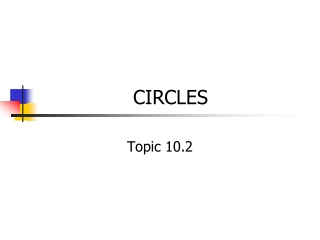# CIRCLES - PowerPoint PPT PresentationDownload PresentationCIRCLES

CIRCLES
Download Presentation## CIRCLES

- - - - - - - - - - - - - - - - - - - - - - - - - - - E N D - - - - - - - - - - - - - - - - - - - - - - - - - - -
##### Presentation Transcript

1. CIRCLES Topic 10.2

2. Definitions • Circle:The set of all points that are the same distance from the center • Radius:a segment whose endpoints are the center and a point on the circle

3. Equation of a Circle

4. Writing the Equation of a Circle • Group x terms together, y-terms together, and move constants to the other side • Complete the square for the x-terms • Remember that whatever you do to one side, you must also do to the other • Complete the square for the y-terms • Remember that whatever you do to one side, you must also do to the other

5. Example: Write the equation and find the center and radius length of : Group terms Complete the square

6. THINK ABOUT IT Find the center, the length of the radius, and write the equation of the circle if the endpoints of a diameter are (-8,2) and (2,0). Center: Use midpoint formula! Length: use distance formula with radius and an endpoint Equation: Put it all together

7. THINK ABOUT IT • Write the equation of a circle whose center is (2, -1) and tangent to x = 6

8. THINK ABOUT IT • Write the equation of a circle who passes through (-2, 3) and is centered at (2, -1)